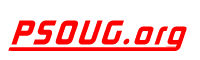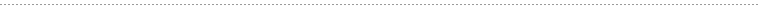Quick Search:Snippet Name: BETWEEN Condition

Description: The BETWEEN condition allows you to retrieve values within a range.

Comment: (none)

Language: PL/SQL
Highlight Mode: PLSQL

```-- The syntax for the BETWEEN condition is:

SELECT columns
FROM tables
WHERE column1 BETWEEN value1 AND value2;

-- This SQL statement will return the records where column1
-- is within the range of value1 and value2 (inclusive). The
-- BETWEEN function can be used in any valid SQL statement -
-- select, insert, update, or delete.

-- Example #1 - Numbers
-- The following is an SQL statement that uses the BETWEEN
-- function:

SELECT *
FROM suppliers
WHERE supplier_id BETWEEN 5000 AND 5010;

-- This would return all rows where the supplier_id is between
-- 5000 and 5010, inclusive. It is equivalent to the following SQL
-- statement:

SELECT *
FROM suppliers
WHERE supplier_id >= 5000
AND supplier_id <= 5010;

-- Example #2 - Dates
-- You can also use the BETWEEN function with dates.

SELECT *
FROM orders
WHERE order_date BETWEEN TO_DATE ('2003/01/01', 'yyyy/mm/dd')
AND TO_DATE ('2003/12/31', 'yyyy/mm/dd');

-- This SQL statement would return all orders where the
-- order_date is between Jan 1, 2003 and Dec 31, 2003
-- (inclusive).

-- It would be equivalent to the following SQL statement:

SELECT *
FROM orders
WHERE order_date >= TO_DATE('2003/01/01', 'yyyy/mm/dd')
AND order_date <= TO_DATE('2003/12/31','yyyy/mm/dd');

-- Example #3 - NOT BETWEEN
-- The BETWEEN function can also be combined with the NOT
-- operator. For example:

SELECT *
FROM suppliers
WHERE supplier_id NOT BETWEEN 5000 AND 5500;

-- This would be equivalent to the following SQL:

SELECT *
FROM suppliers
WHERE supplier_id < 5000
OR supplier_id > 5500;

-- In this example, the result set would exclude all supplier_id
-- values between the range of 5000 and 5500 (inclusive).
```